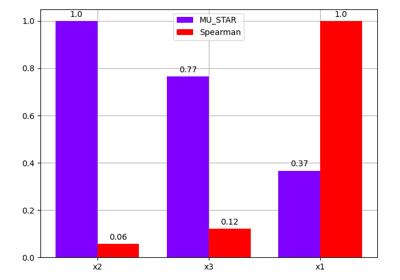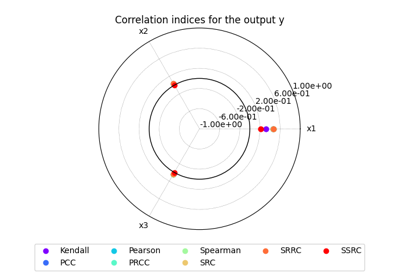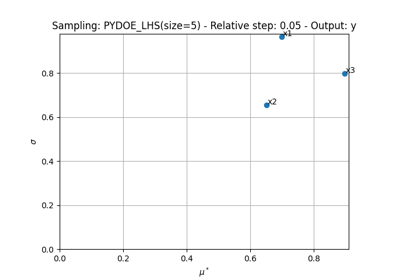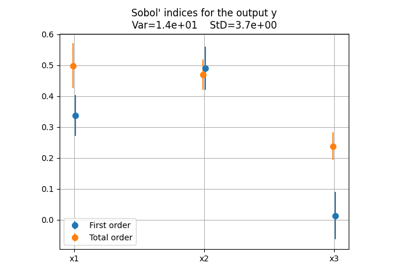gemseo / uncertainty / use_cases / ishigami

# ishigami_space module¶

The uncertain space used in the Ishigami use case.

class gemseo.uncertainty.use_cases.ishigami.ishigami_space.IshigamiSpace[source]

Bases: ParameterSpace

The uncertain space used in the Ishigami use case.

$$X_1,X_2,X_3$$ are independent random variables uniformly distributed between $$-\pi$$ and $$\pi$$.

This uncertain space uses the class SPUniformDistribution.

See [IH90].

Parameters:

name – The name to be given to the design space. If empty, the design space is unnamed.

class DesignVariable(size=1, var_type=_DesignVariableType.FLOAT, l_b=None, u_b=None, value=None)

Bases: NamedTuple

A design variable.

Create new instance of DesignVariable(size, var_type, l_b, u_b, value)

Parameters:
• size (int | None) –

By default it is set to 1.

• var_type (_DesignVariableType | None) –

By default it is set to “float”.

• l_b (ndarray | None) –

• u_b (ndarray | None) –

• value (ndarray | None) –

count(value, /)

Return number of occurrences of value.

index(value, start=0, stop=9223372036854775807, /)

Return first index of value.

Raises ValueError if the value is not present.

l_b: ndarray | None

Alias for field number 2

size: int | None

Alias for field number 0

u_b: ndarray | None

Alias for field number 3

value: ndarray | None

Alias for field number 4

var_type: _DesignVariableType | None

Alias for field number 1

DesignVariableType

alias of _DesignVariableType

Add a random variable from a probability distribution.

Warning

The probability distributions must have the same DISTRIBUTION_FAMILY_ID. For instance, one cannot mix a random variable distributed as a OTUniformDistribution with identifier "OT" and a random variable distributed as a SPNormalDistribution with identifier "SP".

Parameters:
• name (str) – The name of the random variable.

• distribution (str) – The name of a class implementing a probability distribution, e.g. "OTUniformDistribution" or "SPDistribution".

• size (int) –

The dimension of the random variable.

By default it is set to 1.

• **parameters (int | Tuple[str, int, float] | Mapping[str, str | int | float] | None | float) – The parameters of the distribution.

Raises:

ValueError – When mixing probability distributions from different families, e.g. an OTDistribution and a SPDistribution.

Return type:

None

add_variable(name, size=1, var_type=_DesignVariableType.FLOAT, l_b=None, u_b=None, value=None)

Add a variable to the design space.

Parameters:
• name (str) – The name of the variable.

• size (int) –

The size of the variable.

By default it is set to 1.

• var_type (DesignVariableType | Sequence[DesignVariableType]) –

Either the type of the variable or the types of its components.

By default it is set to “float”.

• l_b (float | ndarray | None) – The lower bound of the variable. If None, use $$-\infty$$.

• u_b (float | ndarray | None) – The upper bound of the variable. If None, use $$+\infty$$.

• value (float | ndarray | None) – The default value of the variable. If None, do not use a default value.

Raises:

ValueError – Either if the variable already exists or if the size is not a positive integer.

Return type:

None

array_to_dict(x_array)

Convert a design array into a dictionary indexed by the variables names.

Parameters:

x_array (ndarray) – A design value expressed as a NumPy array.

Returns:

The design value expressed as a dictionary of NumPy arrays.

Return type:
build_composed_distribution(copula=None)

Build the joint probability distribution.

Parameters:

copula (Any | None) – A copula distribution defining the dependency structure between random variables; if None, consider an independent copula.

Return type:

None

check()

Check the state of the design space.

Raises:

ValueError – If the design space is empty.

Return type:

None

check_membership(x_vect, variable_names=None)

Check whether the variables satisfy the design space requirements.

Parameters:
• x_vect (Mapping[str, ndarray] | ndarray) – The values of the variables.

• variable_names (Sequence[str] | None) – The names of the variables. If None, use the names of the variables of the design space.

Raises:

ValueError – Either if the dimension of the values vector is wrong, if the values are not specified as an array or a dictionary, if the values are outside the bounds of the variables or if the component of an integer variable is not an integer.

Return type:

None

clear() None.  Remove all items from D.
compute_samples(n_samples=1, as_dict=False)

Sample the random variables and return the realizations.

Parameters:
• n_samples (int) –

A number of samples.

By default it is set to 1.

• as_dict (bool) –

The type of the returned object. If True, return a dictionary. Otherwise, return an array.

By default it is set to False.

Returns:

The realizations of the random variables, either stored in an array or in a dictionary whose values are the names of the random variables and the values are the evaluations.

Return type:

dict[str, ndarray] | ndarray

dict_to_array(design_values, variable_names=None)

Convert a point as dictionary into an array.

Parameters:
• design_values (dict[str, numpy.ndarray]) – The design point to be converted.

• variable_names (Iterable[str] | None) – The variables to be considered. If None, use the variables of the design space.

Returns:

The point as an array.

Return type:

ndarray

evaluate_cdf(value, inverse=False)

Evaluate the cumulative density function (or its inverse) of each marginal.

Parameters:
• value (dict[str, numpy.ndarray]) – The values of the uncertain variables passed as a dictionary whose keys are the names of the variables.

• inverse (bool) –

The type of function to evaluate. If True, compute the cumulative density function. Otherwise, compute the inverse cumulative density function.

By default it is set to False.

Returns:

A dictionary where the keys are the names of the random variables and the values are the evaluations.

Return type:
extend(other)

Extend the design space with another design space.

Parameters:

other (DesignSpace) – The design space to be appended to the current one.

Return type:

None

extract_deterministic_space()

Define a new DesignSpace from the deterministic variables only.

Returns:

A DesignSpace defined by the deterministic variables only.

Return type:

DesignSpace

extract_uncertain_space(as_design_space=False)

Define a new DesignSpace from the uncertain variables only.

Parameters:

as_design_space (bool) –

If False, return a ParameterSpace containing the original uncertain variables as is; otherwise, return a DesignSpace where the original uncertain variables are made deterministic. In that case, the bounds of a deterministic variable correspond to the limits of the support of the original probability distribution and the current value correspond to its mean.

By default it is set to False.

Returns:

A ParameterSpace defined by the uncertain variables only.

Return type:
filter(keep_variables, copy=False)

Filter the design space to keep a subset of variables.

Parameters:
• keep_variables (str | Iterable[str]) – The names of the variables to be kept.

• copy (bool) –

If True, then a copy of the design space is filtered, otherwise the design space itself is filtered.

By default it is set to False.

Returns:

Either the filtered original design space or a copy.

Raises:

ValueError – If the variable is not in the design space.

Return type:

DesignSpace

filter_dim(variable, keep_dimensions)

Filter the design space to keep a subset of dimensions for a variable.

Parameters:
• variable (str) – The name of the variable.

• keep_dimensions (Iterable[int]) – The dimensions of the variable to be kept, between $$0$$ and $$d-1$$ where $$d$$ is the number of dimensions of the variable.

Returns:

The filtered design space.

Raises:

ValueError – If a dimension is unknown.

Return type:

DesignSpace

Create a design space from a CSV file.

Parameters:
• file_path (str | Path) – The path to the CSV file.

• header (Iterable[str] | None) – The names of the fields saved in the file. If None, read them in the file.

Returns:

The design space defined in the file.

Raises:

ValueError – If the file does not contain the minimal variables in its header.

Return type:

DesignSpace

classmethod from_file(file_path, **options)

Create a design space from a file.

Parameters:
• file_path (str | Path) – The path to the file. If the extension starts with “hdf”, the file will be considered as an HDF file.

• **options – The keyword reading options.

Returns:

The design space defined in the file.

Return type:

DesignSpace

classmethod from_hdf(file_path)

Create a design space from an HDF file.

Parameters:

file_path (str | Path) – The path to the HDF file.

Returns:

The design space defined in the file.

Return type:

DesignSpace

get(k[, d]) D[k] if k in D, else d.  d defaults to None.
get_active_bounds(x_vec=None, tol=1e-08)

Determine which bound constraints of a design value are active.

Parameters:
• x_vec (ndarray | None) – The design value at which to check the bounds. If None, use the current design value.

• tol (float) –

The tolerance of comparison of a scalar with a bound.

By default it is set to 1e-08.

Returns:

Whether the components of the lower and upper bound constraints are active, the first returned value representing the lower bounds and the second one the upper bounds, e.g.

({'x': array(are_x_lower_bounds_active),
'y': array(are_y_lower_bounds_active)},
{'x': array(are_x_upper_bounds_active),
'y': array(are_y_upper_bounds_active)}
)


where:

are_x_lower_bounds_active = [True, False]
are_x_upper_bounds_active = [False, False]
are_y_lower_bounds_active = [False]
are_y_upper_bounds_active = [True]


Return type:

tuple[dict[str, ndarray], dict[str, ndarray]]

get_current_value(variable_names=None, complex_to_real=False, as_dict=False, normalize=False)

Return the current design value.

If the names of the variables are empty then an empty data is returned.

Parameters:
• variable_names (Sequence[str] | None) – The names of the design variables. If None, use all the design variables.

• complex_to_real (bool) –

Whether to cast complex numbers to real ones.

By default it is set to False.

• as_dict (bool) –

Whether to return the current design value as a dictionary of the form {variable_name: variable_value}.

By default it is set to False.

• normalize (bool) –

Whether to normalize the design values in $$[0,1]$$ with the bounds of the variables.

By default it is set to False.

Returns:

The current design value.

Raises:

ValueError – If names in variable_names are not in the design space.

Return type:

ndarray | dict[str, ndarray]

Warning

For performance purposes, get_current_value() does not return a copy of the current value. This means that modifying the returned object will make the DesignSpace inconsistent (the current design value stored as a NumPy array and the current design value stored as a dictionary of NumPy arrays will be different). To modify the returned object without impacting the DesignSpace, you shall copy this object and modify the copy.

To modify the current value, please use set_current_value() or set_current_variable().

get_indexed_var_name(variable_name)

Create the names of the components of a variable.

If the size of the variable is equal to 1, this method returns the name of the variable. Otherwise, it concatenates the name of the variable, the separator DesignSpace.SEP and the index of the component.

Parameters:

variable_name (str) – The name of the variable.

Returns:

The names of the components of the variable.

Return type:

str | list[str]

get_indexed_variable_names()

Create the names of the components of all the variables.

If the size of the variable is equal to 1, this method uses its name. Otherwise, it concatenates the name of the variable, the separator DesignSpace.SEP and the index of the component.

Returns:

The name of the components of all the variables.

Return type:

list[str]

get_lower_bound(name)

Return the lower bound of a variable.

Parameters:

name (str) – The name of the variable.

Returns:

The lower bound of the variable (possibly infinite).

Return type:

ndarray | None

get_lower_bounds(variable_names=None)

Generate an array of the variables’ lower bounds.

Parameters:

variable_names (Sequence[str] | None) – The names of the variables of which the lower bounds are required. If None, use the variables of the design space.

Returns:

The lower bounds of the variables.

Return type:

ndarray

get_pretty_table(fields=None, with_index=False)

Build a tabular view of the design space.

Parameters:
• fields (Sequence[str] | None) – The name of the fields to be exported. If None, export all the fields.

• with_index (bool) –

Whether to show index of names for arrays. This is ignored for scalars.

By default it is set to False.

Returns:

A tabular view of the design space.

Return type:

PrettyTable

get_range(variable)

Return the numerical range of a random variable.

Parameters:

variable (str) – The name of the random variable.

Returns:

The range of the components of the random variable.

Return type:
get_size(name)

Get the size of a variable.

Parameters:

name (str) – The name of the variable.

Returns:

The size of the variable, None if it is not known.

Return type:

int | None

get_support(variable)

Return the mathematical support of a random variable.

Parameters:

variable (str) – The name of the random variable.

Returns:

The support of the components of the random variable.

Return type:
get_tabular_view(decimals=2)

Return a tabular view of the parameter space.

This view contains statistical information.

Parameters:

decimals (int) –

The number of decimals to print.

By default it is set to 2.

Returns:

The tabular view.

Return type:

str

get_type(name)

Return the type of a variable.

Parameters:

name (str) – The name of the variable.

Returns:

The type of the variable, None if it is not known.

Return type:

str | None

get_upper_bound(name)

Return the upper bound of a variable.

Parameters:

name (str) – The name of the variable.

Returns:

The upper bound of the variable (possibly infinite).

Return type:

ndarray | None

get_upper_bounds(variable_names=None)

Generate an array of the variables’ upper bounds.

Parameters:

variable_names (Sequence[str] | None) – The names of the variables of which the upper bounds are required. If None, use the variables of the design space.

Returns:

The upper bounds of the variables.

Return type:

ndarray

get_variables_indexes(variable_names)

Return the indexes of a design array corresponding to the variables names.

Parameters:

variable_names (Iterable[str]) – The names of the variables.

Returns:

The indexes of a design array corresponding to the variables names.

Return type:

ndarray

has_current_value()

Check if each variable has a current value.

Returns:

Whether the current design value is defined for all variables.

Return type:

bool

has_integer_variables()

Check if the design space has at least one integer variable.

Returns:

Whether the design space has at least one integer variable.

Return type:

bool

static init_from_dataset(dataset, groups=None, uncertain=None, copula=None)

Initialize the parameter space from a dataset.

Parameters:
• dataset (Dataset) – The dataset used for the initialization.

• groups (Iterable[str] | None) – The groups of the dataset to be considered. If None, consider all the groups.

• uncertain (Mapping[str, bool] | None) – Whether the variables should be uncertain or not.

• copula (Any) – A name of copula defining the dependency between random variables.

Return type:

ParameterSpace

initialize_missing_current_values()

Initialize the current values of the design variables when missing.

Use:

• the center of the design space when the lower and upper bounds are finite,

• the lower bounds when the upper bounds are infinite,

• the upper bounds when the lower bounds are infinite,

• zero when the lower and upper bounds are infinite.

Return type:

None

is_deterministic(variable)

Check if a variable is deterministic.

Parameters:

variable (str) – The name of the variable.

Returns:

True is the variable is deterministic.

Return type:

bool

is_uncertain(variable)

Check if a variable is uncertain.

Parameters:

variable (str) – The name of the variable.

Returns:

True is the variable is uncertain.

Return type:

bool

items() a set-like object providing a view on D's items
keys() a set-like object providing a view on D's keys

This method is based on the chain rule:

$\frac{df(x)}{dx} = \frac{df(x)}{dx_u}\frac{dx_u}{dx} = \frac{df(x)}{dx_u}\frac{1}{u_b-l_b}$

where $$x_u = \frac{x-l_b}{u_b-l_b}$$ is the normalized input vector, $$x$$ is the unnormalized input vector and $$l_b$$ and $$u_b$$ are the lower and upper bounds of $$x$$.

$\frac{df(x)}{dx_u} = (u_b-l_b)\frac{df(x)}{dx}$

where $$\frac{df(x)}{dx}$$ is the unnormalized one.

Parameters:

g_vect (ndarray) – The gradient to be normalized.

Returns:

Return type:

ndarray

normalize_vect(x_vect, minus_lb=True, use_dist=False, out=None)

Normalize a vector of the parameter space.

If use_dist is True, use the cumulative probability distributions of the random variables to scale the components of the random variables between 0 and 1. Otherwise, use the approach defined in DesignSpace.normalize_vect() with minus_lb.

For the components of the deterministic variables, use the approach defined in DesignSpace.normalize_vect() with minus_lb.

Parameters:
• x_vect (ndarray) – The values of the design variables.

• minus_lb (bool) –

If True, remove the lower bounds at normalization.

By default it is set to True.

• use_dist (bool) –

If True, normalize the components of the random variables with their cumulative probability distributions.

By default it is set to False.

• out (ndarray | None) – The array to store the normalized vector. If None, create a new array.

Returns:

The normalized vector.

Return type:

ndarray

pop(k[, d]) v, remove specified key and return the corresponding value.

If key is not found, d is returned if given, otherwise KeyError is raised.

popitem() (k, v), remove and return some (key, value) pair

as a 2-tuple; but raise KeyError if D is empty.

project_into_bounds(x_c, normalized=False)

Project a vector onto the bounds, using a simple coordinate wise approach.

Parameters:
• normalized (bool) –

If True, then the vector is assumed to be normalized.

By default it is set to False.

• x_c (ndarray) – The vector to be projected onto the bounds.

Returns:

The projected vector.

Return type:

ndarray

remove_variable(name)

Remove a variable from the probability space.

Parameters:

name (str) – The name of the variable.

Return type:

None

rename_variable(current_name, new_name)

Rename a variable.

Parameters:
• current_name (str) – The name of the variable to rename.

• new_name (str) – The new name of the variable.

Return type:

None

round_vect(x_vect, copy=True)

Round the vector where variables are of integer type.

Parameters:
• x_vect (ndarray) – The values to be rounded.

• copy (bool) –

Whether to round a copy of x_vect.

By default it is set to True.

Returns:

The rounded values.

Return type:

ndarray

set_current_value(value)

Set the current design value.

Parameters:

value (ndarray | Mapping[str, ndarray] | OptimizationResult) – The value of the current design.

Raises:
Return type:

None

set_current_variable(name, current_value)

Set the current value of a single variable.

Parameters:
• name (str) – The name of the variable.

• current_value (ndarray) – The current value of the variable.

Return type:

None

set_lower_bound(name, lower_bound)

Set the lower bound of a variable.

Parameters:
• name (str) – The name of the variable.

• lower_bound (ndarray | None) – The value of the lower bound.

Raises:

ValueError – If the variable does not exist.

Return type:

None

set_upper_bound(name, upper_bound)

Set the upper bound of a variable.

Parameters:
• name (str) – The name of the variable.

• upper_bound (ndarray | None) – The value of the upper bound.

Raises:

ValueError – If the variable does not exist.

Return type:

None

setdefault(k[, d]) D.get(k,d), also set D[k]=d if k not in D
to_complex()

Cast the current value to complex.

Return type:

None

Export the design space to a CSV file.

Parameters:
Return type:

None

to_design_space()

Convert the parameter space into a DesignSpace.

The original deterministic variables are kept as is while the original uncertain variables are made deterministic. In that case, the bounds of a deterministic variable correspond to the limits of the support of the original probability distribution and the current value correspond to its mean.

Returns:

A DesignSpace where all original variables are made deterministic.

Return type:

DesignSpace

to_file(file_path, **options)

Save the design space.

Parameters:
• file_path (str | Path) – The file path to save the design space. If the extension starts with “hdf”, the design space will be saved in an HDF file.

• **options – The keyword reading options.

to_hdf(file_path, append=False)

Export the design space to an HDF file.

Parameters:
• file_path (str | Path) – The path to the file to export the design space.

• append (bool) –

If True, appends the data in the file.

By default it is set to False.

Return type:

None

transform_vect(vector, out=None)

Map a point of the design space to a vector with components in $$[0,1]$$.

Parameters:
• vector (ndarray) – A point of the design space.

• out (ndarray | None) – The array to store the transformed vector. If None, create a new array.

Returns:

A vector with components in $$[0,1]$$.

Return type:

ndarray

This method is based on the chain rule:

$\frac{df(x)}{dx} = \frac{df(x)}{dx_u}\frac{dx_u}{dx} = \frac{df(x)}{dx_u}\frac{1}{u_b-l_b}$

where $$x_u = \frac{x-l_b}{u_b-l_b}$$ is the normalized input vector, $$x$$ is the unnormalized input vector, $$\frac{df(x)}{dx_u}$$ is the unnormalized gradient $$\frac{df(x)}{dx}$$ is the normalized one, and $$l_b$$ and $$u_b$$ are the lower and upper bounds of $$x$$.

Parameters:

g_vect (ndarray) – The gradient to be unnormalized.

Returns:

Return type:

ndarray

unnormalize_vect(x_vect, minus_lb=True, no_check=False, use_dist=False, out=None)

Unnormalize a normalized vector of the parameter space.

If use_dist is True, use the inverse cumulative probability distributions of the random variables to unscale the components of the random variables. Otherwise, use the approach defined in DesignSpace.unnormalize_vect() with minus_lb and no_check.

For the components of the deterministic variables, use the approach defined in DesignSpace.unnormalize_vect() with minus_lb and no_check.

Parameters:
• x_vect (ndarray) – The values of the design variables.

• minus_lb (bool) –

Whether to remove the lower bounds at normalization.

By default it is set to True.

• no_check (bool) –

Whether to check if the components are in $$[0,1]$$.

By default it is set to False.

• use_dist (bool) –

Whether to unnormalize the components of the random variables with their inverse cumulative probability distributions.

By default it is set to False.

• out (ndarray | None) – The array to store the unnormalized vector. If None, create a new array.

Returns:

The unnormalized vector.

Return type:

ndarray

untransform_vect(vector, no_check=False, out=None)

Map a vector with components in $$[0,1]$$ to the design space.

Parameters:
• vector (ndarray) – A vector with components in $$[0,1]$$.

• no_check (bool) –

Whether to check if the components are in $$[0,1]$$.

By default it is set to False.

• out (ndarray | None) – The array to store the untransformed vector. If None, create a new array.

Returns:

A point of the variables space.

Return type:

ndarray

update([E, ]**F) None.  Update D from mapping/iterable E and F.

If E present and has a .keys() method, does: for k in E: D[k] = E[k] If E present and lacks .keys() method, does: for (k, v) in E: D[k] = v In either case, this is followed by: for k, v in F.items(): D[k] = v

values() an object providing a view on D's values
DESIGN_SPACE_GROUP = 'design_space'
LB_GROUP = 'l_b'
MINIMAL_FIELDS = ['name', 'lower_bound', 'upper_bound']
NAMES_GROUP = 'names'
NAME_GROUP = 'name'
SEP = '!'
SIZE_GROUP = 'size'
TABLE_NAMES = ['name', 'lower_bound', 'value', 'upper_bound', 'type']
UB_GROUP = 'u_b'
VALUE_GROUP = 'value'
VAR_TYPE_GROUP = 'var_type'
property deterministic_variables: list[str]

The deterministic variables.

dimension: int

The total dimension of the space, corresponding to the sum of the sizes of the variables.

distribution: ComposedDistribution

The joint probability distribution of the uncertain variables.

distributions: dict[str, Distribution]

The marginal probability distributions of the uncertain variables.

name: str | None

The name of the space.

normalize: dict[str, ndarray]

The normalization policies of the variables components indexed by the variables names; if True, the component can be normalized.

uncertain_variables: list[str]

The names of the uncertain variables.

variable_names: list[str]

The names of the variables.

variable_sizes: dict[str, int]

The sizes of the variables.

variable_types: dict[str, ndarray]

The types of the variables components, which can be any DesignSpace.DesignVariableType.

## Examples using IshigamiSpace¶Comparing sensitivity indices

Comparing sensitivity indicesCorrelation analysis

Correlation analysisMorris analysis

Morris analysisSobol’ analysis

Sobol' analysis